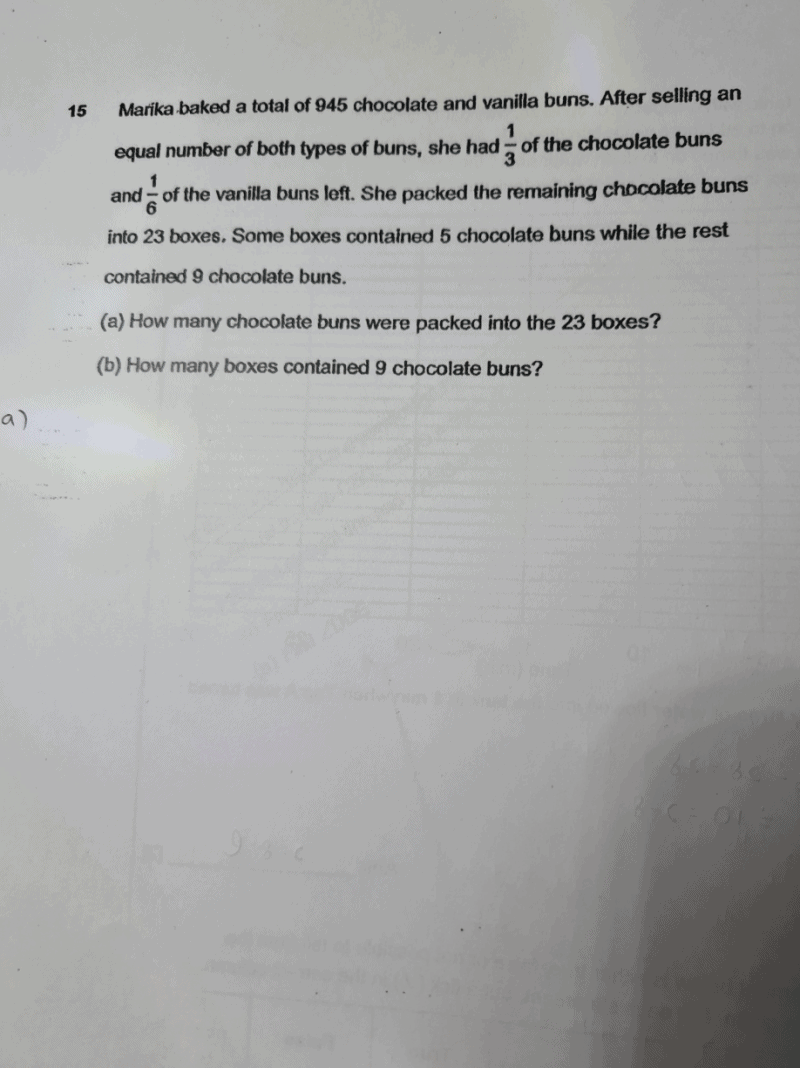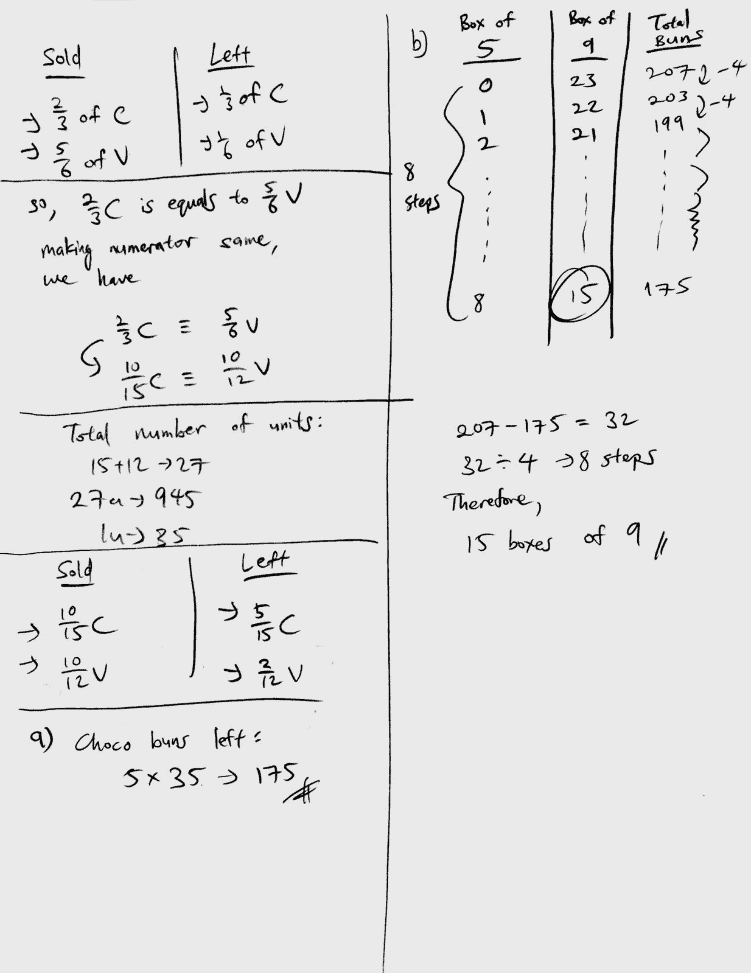# QuestionCan anyone help with this question please. ThanksHi Rachy95, let me try to help you out with this question. Ill provide some explanation along with my solutions.

This question consists of 2 concepts in one.

To solve part a), we use Equal Fractions concept. We use Assumption Method for part b).

Upon reading the question, we learn that Marika sold an equal number of both type of buns. That means, the quantity of 2/3 of the chocolate buns, and 5/6 of the vanilla buns were equal, hence the name of the concept; Equal Fractions. When dealing with Equal Fractions, we “make the numerator for both the fractions same”.

We end up with 10/15 and 10/12 respectively.

We also know that, in general, denominators represents the total. Hence over here, there are a total of 15 units of Chocolate and 12 units of Vanilla. That also makes a total of 27 units for both flavors.

These 27 units represent 945 buns (given in the first sentence).

Therefore, 1 unit represents 35.

There are a 5 units of chocolate buns left, so therefore, 5 units is 175.

To solve part b), we use the Assumption Method. This concept and method for solving sums is very commonly taught in schools. It is like the Guess-and-Check method, except it is organised. Think of it as and ‘upgraded’ version of Guess-and-Check!

To interpret the diagram shown for part b),

If there are 0 boxes of 5 and 23 boxes of 9, there will be a total of 207 buns.

If there is 1 box of 5 and 22 boxes of 9, there will be 203.

2 boxes of 5 and 21 boxes of 9 gives, 199.

Notice that each step we take, we add 1 box to box of 5 and subtract 1 box from box of 9.

We keep total boxes at 23 total, as the question states so.

Each step we take, the total number of buns also reduces by 4.

We want to reduce the total to 175 buns, as part a) states so.

We find out that we take 8 steps, starting from the very beginning to achieve 175 buns.

That means, there is a total of 8 boxes of 5 and 15 boxes of 9.

The 2 concepts, Equal Fractions and Assumption Method goes quite in-depth. Will be happy to share more with you if needed. The problem sums involving these 2 concepts are commonly tested and contain a huge deal of marks in examination papers.

Hope this solution provided above helps!

Feel free to PM or text me (:

http://www.futureachievers.com.sg

9114 3397